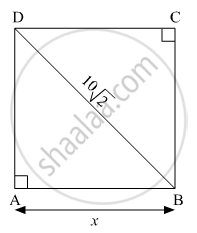Advertisement Remove all ads

# Some Question and Their Alternative Answer Are Given. Select the Correct Alternative. Find Perimeter of a Square If Its Diagonal is 10 √ 2 - Geometry

MCQ

Some question and their alternative answer are given. Select the correct alternative.

Find perimeter of a square if its diagonal is $10\sqrt{2}$

#### Options

• 10 cm

•  $40\sqrt{2}$cm

• 20 cm

• 40 cm

Advertisement Remove all ads

#### SolutionIt is given that ABCD is a square.

∴ AB = BC = CD = DA = x (say)

According to Pythagoras theorem, in ∆ABD

${\text{AB}}^2 + {\text{AD}}^2 = {\text{BD}}^2$
$\Rightarrow x^2 + x^2 = \left( 10\sqrt{2} \right)^2$
$\Rightarrow 2 x^2 = 200$
$\Rightarrow x^2 = 100$
$\Rightarrow x = \sqrt{100}$
$\Rightarrow x = 10 \text{cm}$

Hence, the side of the square is 10 cm.

Now,
Perimeter of a square = $4 \times \left( side \right)$

=$4 \times x$

=$4 \times 10$

=$40$

Hence, the correct option is 40 cm.

Concept: Apollonius Theorem
Is there an error in this question or solution?
Advertisement Remove all ads

#### APPEARS IN

Balbharati Mathematics 2 Geometry 10th Standard SSC Maharashtra State Board
Chapter 2 Pythagoras Theorem
Problem Set 2 | Q 1.5 | Page 43
Advertisement Remove all ads
Advertisement Remove all ads
Share
Notifications

View all notifications

Forgot password?